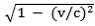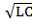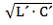# ВЛИЯНИЕ РЕЛЯТИВИСТСКОГО ЗАМЕДЛЕНИЯ ВРЕМЕНИ НА ДИНАМИКУ И УСТОЙЧИВОСТЬ ЛИНЕЙНЫХ СИСТЕМ

Сучилин Владимир Александрович
Transoffice-Information GbR
Фильдерштадт (Германия), Технический директор

Аннотация
В статье показано, что в результате релятивистского замедления времени коэффициенты обыкновенных дифференциальных уравнений, описывающих динамику линейной системы с сосредоточенными параметрами, изменяют свои значения. Это приводит, прежде всего, к изменению динамических характеристик и показателей устойчивости системы, связанных с этими коэффициентами. С другой стороны, поскольку последние определяются элементами системы, то эти изменения, по существу, можно рассматривать с точки зрения эквивалентной модификации параметров самих элементов. В качестве примера рассмотрено влияние релятивистского замедления времени на динамические характеристики колебательного звена. Показано, что замедление времени не нарушает устойчивости линейной системы, хотя и сокращает ее запас устойчивости.

# RELATIVISTIC TIME DILATION IMPACT ON DYNAMICS AND STABILITY OF LINEAR SYSTEMS

Transoffice-Information GbR

Abstract
It is shown, that as a result of the relativistic time dilation the coefficients of ordinary differential equations describing the dynamics of a linear system with lumped parameters change their values. This entails the change in system characteristics and stability indicators associated with these coefficients. On the other hand, since the latter are determined by elements of the system, this change in itself can be treated equivalent to the change in parameters of these elements. As example, the relativistic time dilation impact on dynamic characteristics of oscillation circuit is considered. It is shown that the time dilation does not make the system unstable though reduces its stability margin.

Библиографическая ссылка на статью:
Сучилин В.А. Relativistic Time Dilation Impact on Dynamics and Stability of Linear Systems // Современные научные исследования и инновации. 2018. № 2 [Электронный ресурс]. URL: https://web.snauka.ru/issues/2018/02/85729 (дата обращения: 02.06.2023).

Introduction

The relativistic time dilation is one of the fundamental issues of Einstein’s special relativity, which has to do with inertial reference systems (IRS) travelling in the homogeneous and isotropic space with a steady velocity . At the same time, as opposed to the stationary IRS which is associated with observer all other inertial systems are defined as moving IRS. In the following, all the notations with acute accent (´) will be related to the moving IRS. The key quotient of the relativistic time dilation (named Lorentz factor ) is given by

γ = 1 /(1)

where

v – relative velocity of the moving IRS

c – velocity of light in free space.

Then, basically, relativistic time dilation is expressed by the equality

t´ = γ t , γ > 1 (2)

where

t´ – time interval measured in the moving IRS

t – corresponding time interval measured in the stationary IRS

Until now, the relativistic time dilation has been tested and has received confirmation through physical experiments . The human perceiving of the time dilation was studied in . In technical context, the impact of the relativistic time dilation on signals and systems has been discussed in .

Dynamics of linear system in the moving IRS

The dynamics of the linear system configured in the stationary IRS is described by the ordinary differential equation with constant coefficients 

anx(n)(t) + an-1x(n-1)(t) + … + aоx(t) = f(t) (3)

where

a- coefficients associated with the system parameters in the stationary IRS

x(t) – system response to the input action f(t)

Representing equality (2) in differential form we obtain

dt´ = γ dt (4)

This clearly implies

dt = dt´/ γ (5)

Then substituting (5) in (3) one immediately gets the differential equation for the moving IRS in the form

γnanx(n)(t´) + γn-1an-1x(n-1)(t´) + … + aоx(t´) = f(t´) (6)

or otherwise

an´x(n)(t´) + an-1´x(n-1)(t´) + … + aо´x(t´) = f(t´) (7)

where

ak´ = γkak , k = 0 … n (8)

Thus, the relativistic time dilation in the moving IRS leads to the differential equation, which coefficients are modified by the Lorentz factor γ. At the same time, these coefficients are associated in a certain way with the elements of the analyzed system. Therefore, along with the change in the characteristics of the system, one can also speak about a change of parameters of its elements due to the relativistic time dilation. This will also be shown in the Example 1 below.

Stability of a linear system placed in the moving IRS

Consider a stable linear system configured in the stationary IRS. The question is how the relativistic time dilation affects the stability of this system to be placed in a moving IRS. As well-known, for stability of a linear system it is necessary and sufficient that all roots of the characteristic equation of the system should have the negative real parts .

Represent the characteristic polynomial associated with the equation (3) as a product

P(s) = an (γs – so) (γs – s1) … (γs – sn-1) (9)

where so, …, sn-1 are the roots (generally, complex) of the characteristic equation.

Next, the polynomial (9) can be written in the form

P(s) = an´ (s – so´) (s – s1´) … (s – sn-1´) (10)

where an´ = γnaand sk´ = sγ.

Then for all roots of the polynomial (10)

Re(sk`) = Re(sk) / γ , k=0, …, n-1 (11)

Since by definition γ is positive the sign in (11) is retained. Therefore, a stable linear system retains its stability despite the relativistic time dilation impact.

On the other hand, since γ > 1 the equality (11) implies

Re(sk`) < Re(sk) , k=0, …, n-1 (12)

That means that the stability margin of the analyzed system decreases. In other words, if the stability margin of the linear system configured in the stationary IRS is given through the quotient η0, then in the moving IRS it will be equal η0/γ.

Example 1

Consider the second-order system with its differential equation:

x(2)(t) + a1x(1)(t) + aox(t) = γ2 , γ > 1 (13)

where a1=2δ and ao=ω2 are attenuation coefficient and resonance frequency accordingly.

Then in view of (7) and (8) we obtain

x(2)(t´) + 2δ´x(1)(t´) + ω´2x(t´) = 1 (14)

where in view of (6) δ´ = δ/γ and ω´ = ω/γ.

In particular, in case of series RLC circuits : δ = R/L and ω = 1/.

In the moving IRS these parameters are given by

δ´ = R / γL (15-a)

ω´ = 1 / γ(15-b)

Here, δ´ plays also the role of the stability margin. Thus according to equality (15-a) stability of the circuit decreases.

On the other hand 1/δ is considered as response time Δ of RLC circuit , and the latter in regard to (15-a) increases with the factor γ. Moreover, the same effect follows from (15-b) for oscillation cycle. Hence, in the moving IRS response time and oscillation cycle are given by

Δ´ = γ·Δ (16-a)

T´ = γ·2π(16-b)

At the same time

T = 2π(17)

Then in view of (16-b) and (17) we obtain

T´ = γ·T (18)

Hence, the oscillation period in the moving IRS increases with factor γ. This is in full accordance with the equality (2) for the relativistic time dilation.

Moreover, the equality (15-a) can be written as

δ´ = R / L´ (19)

where

L´ = γ·L (20)

On the other hand in the moving IRS

T´ = 2π(21)Then under consideration of (19), comparing (16-b) and (21) we obtain

C´= γ·C (22)

Hence, formally it can be admitted that in an oscillation circuit to be placed in the moving IRS the values of C and L are increasing in accordance with (20) and (22). Note that the physical aspect of this statement is beyond the scope of this paper.

Example 2

Consider the characteristic equation of a stable three-order linear system

as3 + as2 + as1 + aо = 0 (23)

According to Hurwitz’s criterion , the coefficients in (23) are positive and also

a1 a2 – ao a3 > 0 (24)Then in case of the system placed in the moving IRS the polynomial (23) takes the form

a3´ s3 + a2´ s2 + a1´ s1 + aо´ = 0 (25)

where (in accordance with (7) by γ >1) all coefficients stay positive.

Next, using (8) and (24) we obtain

a1´ a2´ – ao´ a3´ = γ(a1 a2 – ao a3) > 0 (26)

Therefore, the system retains its stability in the moving IRS despite the relativistic time dilation impact.

Discussion

As it was shown above, the relativistic time dilation leads to a change in parameters of the system without loss of its stability. The stationarity of the system is retained by definition since it is about inertial reference. In this case, the Lorentz factor and coefficients of the differential equation describing the system remain constant. Moreover, this effect may turn out to be more or less significant, depending on the value of the Lorentz factor. For example, if in the coming decades the speeds in the space field can reach about 0.01% of the velocity of light in free space, the values ​​of the Lorentz factor will remain less than 1.000000005, which in many cases entails that the time dilation impact remains within the limits of measurement error or manufacturing tolerance. Nevertheless, by the applications which require rather precise measurements such as GPS , the relativistic time dilation requires an appropriate fine-tuning of the equipment already at the current stage of technical development.

Conclusions

As shown above, owing to the relativistic time dilation impact on linear system the coefficients of its differential equation change their values in the moving IRS. This leads, first of all, to the change in system characteristics which are associated with these coefficients (such as, attenuation coefficient or response time). On the other hand, these changes can be formally treated equivalent to the modification of parameters of individual elements. And finally, the time the relativistic time dilation impact does not make the system unstable though reduces its stability margin.

References
1. Forshaw Jeffrey, Smith Gavin. Dynamics and relativity. John Wiley & Sons: 2014: 344 p.
2. Bailey J., Borer K., Combley F., Drumm H., et al. Test of relativistic time dilation with fast optical atomic clocks at different velocities. Nature Physics 3, 861–864 (2007)
3. Kanai R., et al. Time dilation in dynamic visual display. Journal of Vision 6, 1421-1430 (2006)
4. Soutchilin V. A. Relativistic Approach to Signals and Systems // Современные научные исследования и инновации. 2017. № 11 [Электронный ресурс. URL: http://web.snauka.ru/issues/2017/11/84761
5. Попов В. Л. Теория линейных систем регулирования и управления. М.: Наука, 1989. 304 с.
6. Атабеков Г. И. Теоретические основы электротехники. Линейные электрические цепи. М.: Лань, 2008: 591 с.
7. Ashby Neil. Relativity in the Global Positioning System. Living Reviews in Relativity. Department of Physics, University of Colorado, Boulder, Colorado 80309-0390 USA.